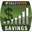# Savings Calculator

 Years to Invest Starting Balance \$ Interest Rate % Periodic Deposit \$ Deposit Frequency AnnuallySemi-AnnuallyQuarterlyBi-MonthlyMonthlySemi-MonthlyBi-WeeklyWeekly Extra AnnualDeposit \$

## DESCRIPTIONThis simple savings calculator estimates the future value of your savings after a number of years making regular deposits. It assumes a fixed rate of return, but the actual interest rate may change over time, depending on the type of investment and market fluctuations. The earnings are compounded according to the deposit frequency that you choose, and it is assumed that the deposits are made at the end of each period.

Savings Bond Calculator - To use this calculator for estimating the future value of a savings bond, set the Periodic Deposit and Extra Annual Deposit to zero and the Deposit Frequency to Semi-Annually (or other compound frequency depending on the type of bond).

Note: This calculator does not include taxes on interest earned, dividends, or capital gains.

Years to Invest - Enter the number of years that you will be investing your money.

Starting Balance - The current value of your savings or your initial investment amount.

Interest Rate - This is the estimated annual interest rate that you expect to earn. Actual interest rates may change over time. Interest is compounded according to the Deposit Frequency that you choose.

Periodic Deposit - This is the amount the you will invest at regular intervals, based on the Deposit Frequency that you choose.

Deposit Frequency - Deposits are made at the end of each period (e.g. monthly, weekly, ...). The compound frequency is assumed to be the same as the deposit frequency.

Extra Annual Deposit - The additional deposit or investment that you plan to make at the end of each year.

## More Financial Calculators

If you use Excel, try the Savings Interest Calculator for Excel

Disclaimer: This calculator and information is provided for illustrative and educational use only. The results are only estimates, so do not make financial decisions based on the results of this calculator.